OK, so what do we do it the numbers are too big for a number line?

How do we know if the answer will be positive or negative?

Let's look at one we did with small numbers: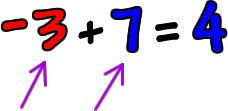Look at these numbers without their signs:  3 and 7

Which is bigger?  The 7.

So, the answer will have that guy's sign... which is positive!

Look at this one: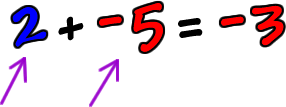Look at these and ignore the signs:  2 and 5

Who's bigger?  The 5.

What's his sign?  Negative.  So, the answer is negative!

Let's do one with bigger numbers: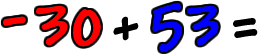First, will the answer be positive or negative?

30 and 53... 53 wins... The answer is positive.

Now, we need to get the number part of the answer.

Here's what you do:

 1 Ignore the signs 2 Take the biggest numberand subtract off the smaller one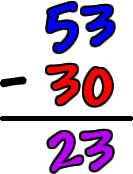And we leave it positive.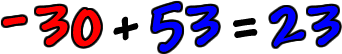Hey, don't panic!  This is easy and you'll get used to it.  We just need to do more of them!